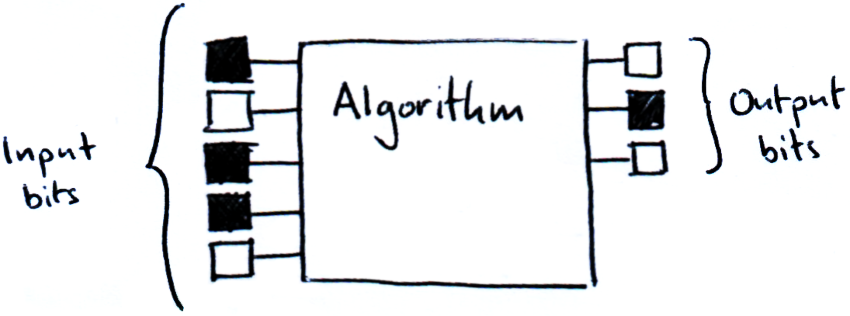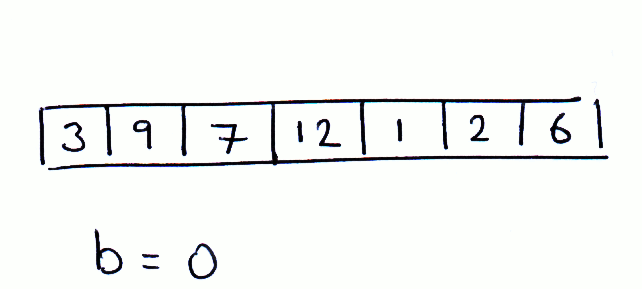# Preliminary Computer Science

## Algorithms

Here we introduce the concept of an algorithm and explain how algorithm performance is measured with some simple examples.

### Contents

1. What is an Algorithm?
2. Algorithm Complexity

## What is an Algorithm?

An algorithm is a very specific set of instructions that can be carried out by a computer. There can be no ambiguity in an algorithm since a computer is not able to think for itself. We generally start with an input state which in our case will be a sequence of bits, perform some operations on these bits and return an output state (again, in bits).A very simple example of an algorithm could be one that finds the largest entry in a list of positive numbers:

### Example: Finding the Largest Number in a List

We use the variable 'b' to keep track of the largest number.

1. Start
2. Set ‘b’ to 0
3. For each number, ‘x’, in our list:
• if ‘x’ is bigger than b, set ‘b’ to the value of ‘x’
4. Return ‘b’ as the biggest number in the list.In this example, the input is the list of numbers and the output is the largest number in the list. Note that the running time of the algorithm is proportional the size of the input list.

## Algorithm Complexity

We measure the efficiency of an algorithm by how the running time grows with the number of input bits (n). For example:

• An algorithm that checks whether a number is even or odd must only check the rightmost bit, so this algorithm takes the same number of steps to run regardless of how big the number is. This is O(1) in big-O-notation.
• An algorithm that counts the number of 1s in a binary sequence will grow proportionally to our input, this is O(n). If we look at our largest-number-in-a-list algorithm from before, we can see that the number of steps in our algorithm grows proportionally to the size of our list. Since our list is made from bits, this is O(n).
• An algorithm that counts through each possible state our bits can be in will grow exponentially with the number of bits we have, this is O(2n).

We group these different rates of growth into different ‘complexity classes’, bear in mind ‘complexity’ of an algorithm is not to do with how complicated it is to humans, but how many steps it takes for a computer.

But why do we measure algorithms like this? Different computers run at different speeds, but with a high enough number of bits we know that an algorithm from a lower complexity class will always outperform an algorithm from a higher complexity class.

Example:

We have an algorithm (we will call this algorithm ‘A’), that grows proportionally to 3n × 2n. We say this algorithm runs in ‘exponential time’, and runs in O(2n). Note that we have ignored the polynomial factor of 3n because it is negligible compared to the huge factor of 2n.

Now let’s compare this to another algorithm from a lower time complexity: Say we have another algorithm (B) that grows proportionally to 5n10, we say this algorithm runs in ‘polynomial time’ and has complexity O(n10), note again that we ignore the constant factor of 5 since this is insignificant compared to our polynomial factor n10.

For small problems (n < 50), our exponential time algorithm (A) seems to outperform the polynomial time algorithm (B), but by the time n grows to 60, our polynomial time algorithm (B) is 100 times faster. If we go further and look at a problem with 100 bits, algorithm B is 1000000000000 times faster.

Now if we were only working with small values for n then it might make sense to use algorithm A, but this is highly problem specific and depends on many factors such as the hardware you are using.

## Big-O-Notation

We have seen big-O-notation in use, but we will address it directly. When looking at the rate at which an algorithm grows with n, we often omit factors of a lower time complexity, e.g. an algorithm that grows by log(n)×n2 could said to have complexity O(n2), since logarithmic time is lower than polynomial time and has less effect at high n.